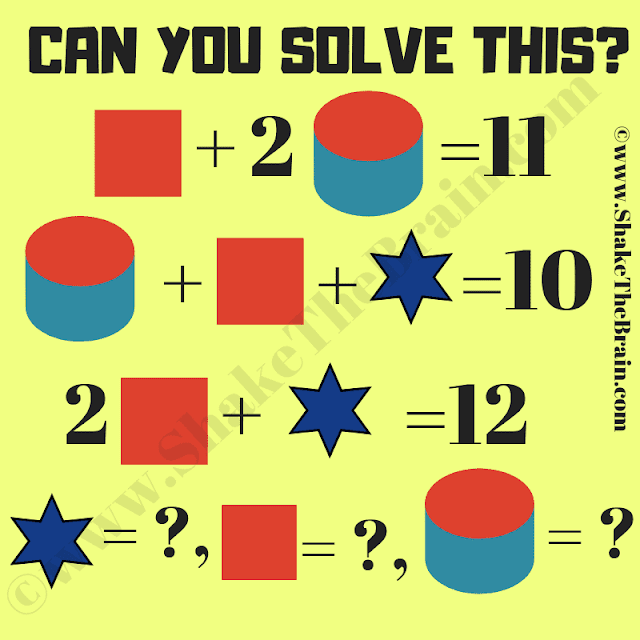Engage with a maths brain teaser: an algebra picture puzzle. Challenge yourself with this captivating mathematical question.

Here is the Maths Picture Puzzle to solve the Pictorial equations.  In this Maths Picture Puzzle, you are shown some algebraic equations. There are three variables in these equations, Square, Star, and Cylinder. Can you find the value of each of the variables in these Maths equations?Can you solve this math picture puzzle?

The answer to this "Maths Picture Puzzle", can be viewed by clicking the answer button.

#### 1 comment:

toddg said...

Except that line 1 square (5) + 2 + cylinder (3) = 10, not 11.